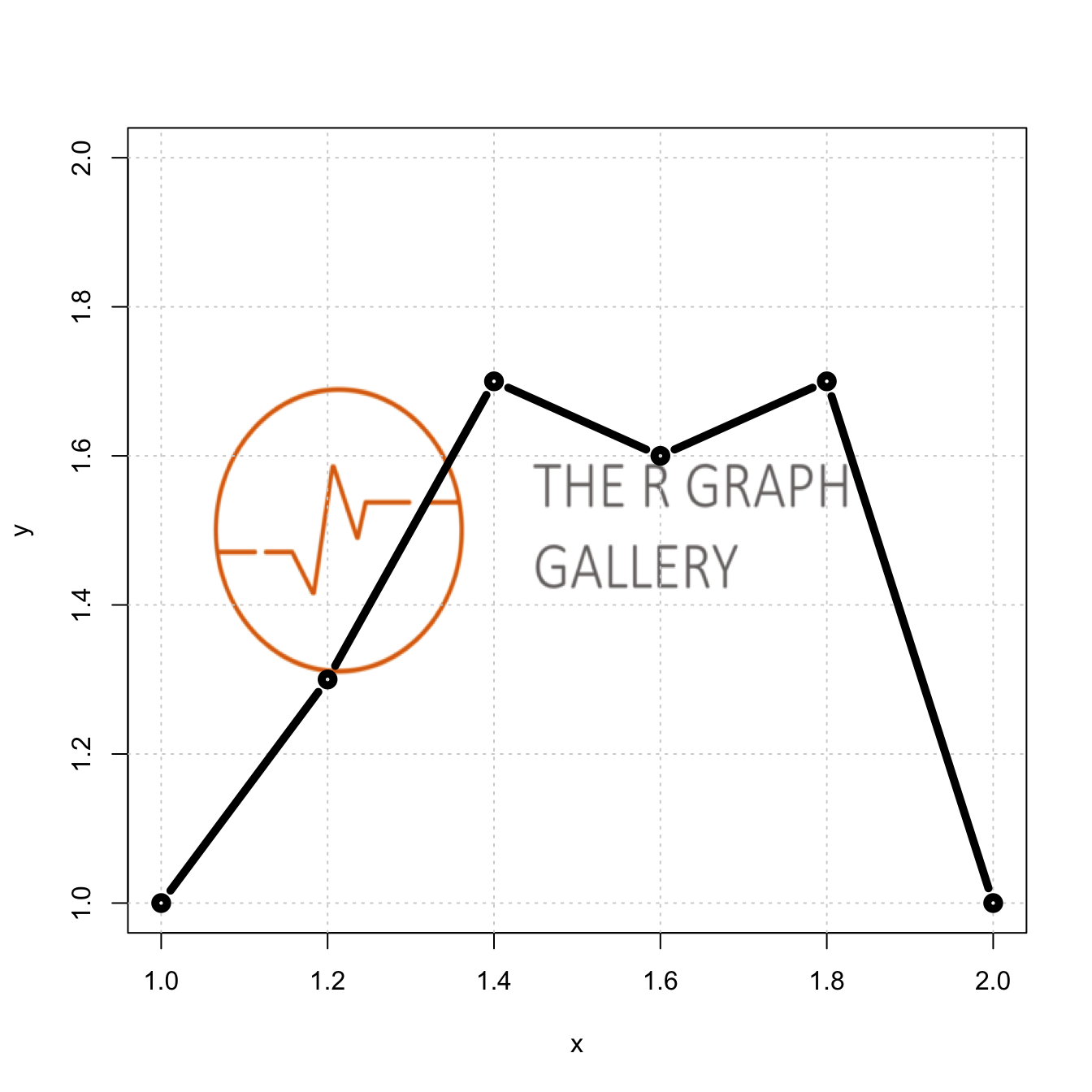# Plot with an image as background

This post explains how to build a chart with an image in the background. It is done thanks to the `jpeg` R package.

First of all, find an image. If the image is on the web, you can download it with `download.file()`.

Then, load the image on R with the `png` library nd the `readPNG()` function. Last, it is the `rasterImage()` function that allows to put it on the background.

Note: if you have a jpeg file. Use `library(jpeg)` instead, and use the `readJPG()` function.``````# If you want to show an image coming from the web, first download it with R:
#Else, just place the image in the current directory

# Charge the image as an R object with the "JPEG" package
library(jpeg)
library(png)

# Set up a plot area with no plot
plot(1:2, type='n', main="", xlab="x", ylab="y")

# Get the plot information so the image will fill the plot box, and draw it
lim <- par()
rasterImage(my_image,
xleft=1, xright=2,
ybottom=1.3, ytop=1.7)
grid()

lines(
x=c(1, 1.2, 1.4, 1.6, 1.8, 2.0),
y=c(1, 1.3, 1.7, 1.6, 1.7, 1.0),
type="b", lwd=5, col="black")``````

Related chart types

## Contact

This document is a work by Yan Holtz. Any feedback is highly encouraged. You can fill an issue on Github, drop me a message on Twitter, or send an email pasting yan.holtz.data with gmail.com.